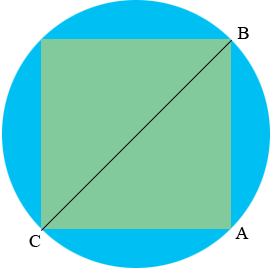SEARCH HOMEMath Central Quandaries & QueriesQuestion from Bill: bill wants to put a 2 foot square peg into a round hole What must be the circumference of the hole?Hi Bill,

Here is my diagram of a green square peg on a round hole.By the symmetry $BC$ is a diameter of the circle. The angle at $A$ is a right angle. $|CA| = |AD| = 2$ feet. What is the length of $BC?$

PennyMath Central is supported by the University of Regina and The Pacific Institute for the Mathematical Sciences.